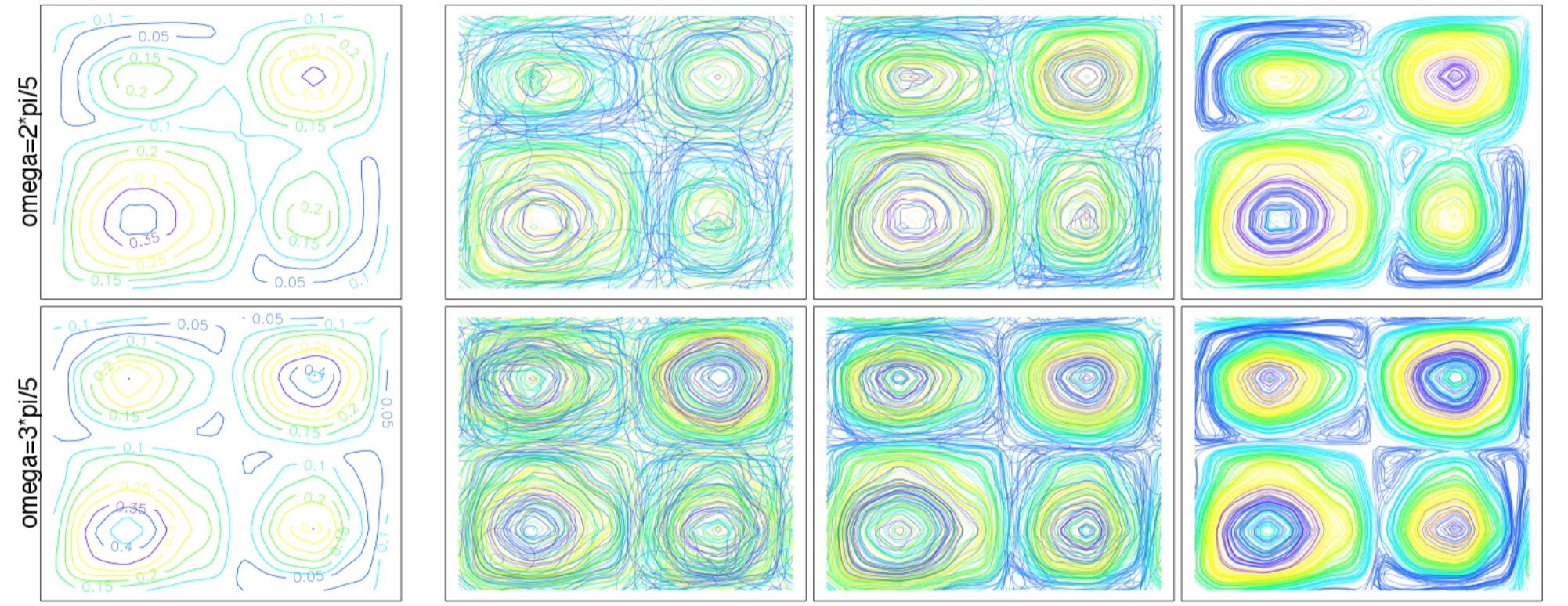Fourier Analysis of Functional Time Series with Applications to DNA Dynamics

Abstract

This work is about time series of functional data (functional time series), and consists of three main parts. In the first part (Chapter 2), we develop a doubly spectral decomposition for functional time series that generalizes the Karhunen–Loève expansion. In the second part (Chapter 3), we develop the theory of estimation for the spectral density operators, which are the main tool involved in the doubly spectral decomposition. The third part (Chapter 4) is concerned with the problem of understanding and comparing the dynamics of DNA. It proposes a methodology for comparing the dynamics of DNA minicircles that are vibrating in solution, using tools developed in this thesis. In the first part, we develop a doubly spectral representation of a stationary functional time series that generalizes the Karhunen–Loève expansion to the functional time series setting. The representation decomposes the time series into an integral of uncorrelated frequency components (Cramér representation), each of which is in turn expanded in a Karhunen-Loève series, thus yielding a Cramér–Karhunen–Loève decomposition of the series. The construction is based on the spectral density operators—whose Fourier coefficients are the lag-t autocovariance operators—which characterise the second-order dynamics of the process. The spectral density operators are the functional analogues of the spectral density matrices, whose eigenvalues and eigenfunctions at different frequencies provide the building blocks of the representation. By truncating the representation at a finite level, we obtain a harmonic principal component analysis of the time series, an optimal finite dimensional reduction of the time series that captures both the temporal dynamics of the process, and the within-curve dynamics, and dominates functional PCA. The proofs rely on the construction of a stochastic integral of operator-valued functions, whose construction is similar to that of the Itô integral. In practice, the spectral density operators are unknown. In the second part, we therefore develop the basic theory of a frequency domain framework for drawing statistical inferences on the spectral density operators of a stationary functional time series. Our main tool is the functional Discrete Fourier Transform(fDFT).We derive an asymptotic Gaussian representation of the fDFT, thus allowing the transformation of the original collection of dependent random functions into a collection of approximately independent complex-valued Gaussian random functions. Our results are then employed in order to construct estimators of the spectral density operators based on smoothed versions of the periodogram kernel, the functional generalisation of the periodogram matrix. The consistency and asymptotic law of these estimators are studied in detail. As immediate consequences, we obtain central limit theorems for the mean and the long-run covariance operator of a stationary functional time series. Our results do not depend on structural modeling assumptions, but only functional versions of classical cumulant mixing conditions. The effect of discrete noisy observations on the consistency of the estimators is studied in a framework general enough to apply to a wide range of smoothing techniques for converting discrete noisy observations into functional data. We also perform a simulation study to assess the finite sample performance of our estimators, and give a discussion of the technical assumptions of our results, and at what cost our weak dependence assumptions could be changed or weakened, and provide examples of processes satisfying the technical assumptions of our asymptotic results. As an application, we consider in the third part the problem of comparing the dynamics of the trajectories of two DNA minicircles that are vibrating in solution, which are obtained via Molecular Dynamics simulations. The approach we take is to view and compare the dynamics through their spectral density operators, which contain the entire second-order structure of the trajectories. As a first step, we compare the spectral density operators of the two DNA minicircles using a new test we develop, which allows us to compare the spectral density operators at a fixed frequencies. Using multiple testing procedures, we are able to localize in frequencies the differences in spectral density operators of the two DNA minicircles, while controlling a type-I error, and conduct numerical simulations to assess the performance of our method. We further investigate the differences between the two minicircles by comparing their spectral density operators within frequencies. This allows us to localize their differences both in frequencies and on the minicircles, while controlling the averaged false discovery rate over the selected frequencies. Our methodology is general enough to be applied to the comparison of the dynamics of any pair of stationary functional time series.

Type
Publication
EPFL PhD Thesis